Maths
Study Material

# Properties of Determinants | Examples

Determinants are the quantity(scalar) attained by the sum of products of the elements of a square matrix according to a specific rule

8 minutes long
Posted by Kunduz Tutor, 19/10/2021Hesap Oluştur

Got stuck on homework? Get your step-by-step solutions from real tutors in minutes! 24/7. Unlimited.

Determinants are the quantity(scalar) attained by the sum of products of the elements of a square matrix according to a specific rule. The determinants help us to determine the adjoint and inverse of a matrix. Here, we will learn about properties of determinants.

Author – Ojasvi Chaplot

## Determinant of a Matrix

The word ‘Matrix’ refers to an ordered rectangular arrangement of numbers in rows and columns; which are either real or complex. The elements of a matrix are enclosed in [] or ().

The matrix, say A, is denoted as A = [aij]i×j ; where i, j are positive integers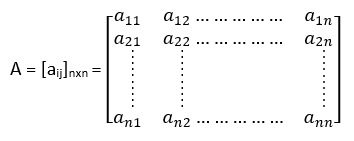The Order of a Matrix, n = Number of Rows x Number of Columns = Number of Elements

A ‘Determinant’ is a particular number or some specific numeric value whose value is obtained by using the square matrix. Along with which the ‘Properties of Determinants’ help us to simplify the evaluation of determinant by obtaining the maximum number of zeros in a row or a column. The elements of a matrix are enclosed in ||.

The determinant of a matrix, say A, is denoted by the following ways: det(A), |A|, det A, or ∆.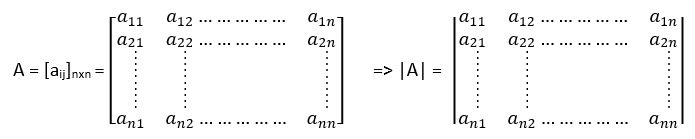For Example – Let A be a square matrix of order 2 such that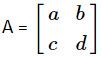Then, the det(A) or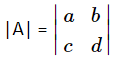= ad – bc.

Similarly, if A is a square matrix of order 3 such that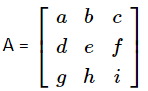Then,

the det(A) or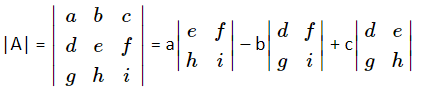a(ei – fh) – b(di – fg) + c(dh – eg) = aei – afh – bdi + bfg + cdh – ceg

This way the determinant of a matrix is calculated. Moreover, you will learn about the properties of determinants by means of which you can solve the determinants of complex problems easily.

## The Basic Properties of Determinants

The basic properties of determinants are stated as follows:

• |In| = 1 ; where Iis the identity matrix of order n.
• |On| = 1 ; where Ois the null or zero matrix of order n.
• |AB| = |A||B| ; where A, B are square matrices of order n.
• |AT| = |A| ; where A is a square matrix of order n.
•  |A-1| = 1/|A| ; where A is a square matrix of order n.
• |kA| = kⁿ|A| ; where k is a constant and A is a square matrix of order n.
• |Aⁿ| = |A|ⁿ ; where A is a square matrix of order n.
• |adj A| =  |A|n-1  ; where A is a square matrix of order n.
• In case of a triangular matrix (either upper or lower), the value of the determinant is equal to the product of its diagonal elements.

## The Standard/Important Properties of Determinants

The standard and important properties of determinants are mentioned below:

Let A be a square matrix such that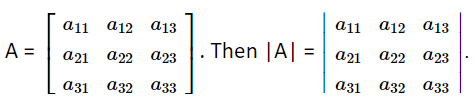## Reflection Properties of Determinants

The reflection property of determinants states that the value of determinant does not change if the rows are transformed into the columns and the columns are transformed into the rows. In simple words, it is said that the value of the determinant remains unchanged if the rows or columns are interchanged or vice versa; i.e. |A| = |AT|.

Let’s say,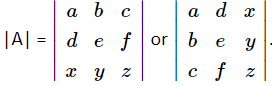. Then, |A| = |AT|

## All-Zero Property of Determinants

The all-zero property of determinants describes that the value of a determinant is 0, if each element of a row (or column) of the determinant is 0.

Let’s say,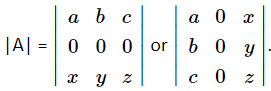. Then, |A| = 0.

## Proportionality (or Repetitive) Properties of Determinants

The proportionality or repetition property of determinants describes that if any two rows (or columns) of a determinant are identical (or proportional), then the value of the determinant is 0.

Let’s say,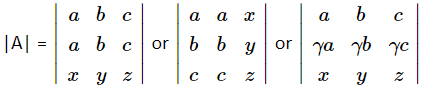;  where γ = constant. Then, |A| = 0.

## Switching Property of Determinants

The switching properties of determinants justifies the point that if any two rows (or columns) of a determinant are interchanged, then the value of determinant changes by a negative sign only.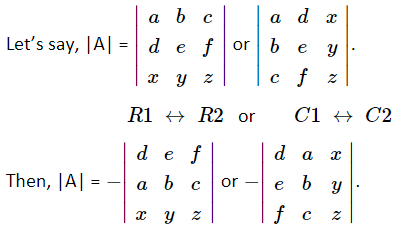## Scalar Multiple Property of Determinants

The scalar multiple property of determinants says that if all the elements of a row (or column) of a determinant are multiplied by a non-zero constant, then the determinant gets multiplied by the same non-zero constant.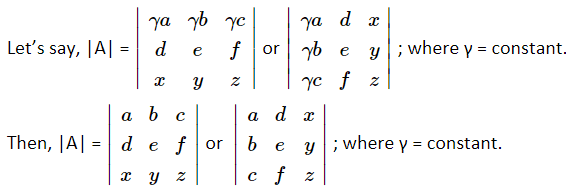## Sum Property of Determinants

The sum property of determinants states that if each element of a row (or column) of a determinant is expressed as the sum of two or more terms, then the determinant can be expressed as the sum of two or more determinants. For example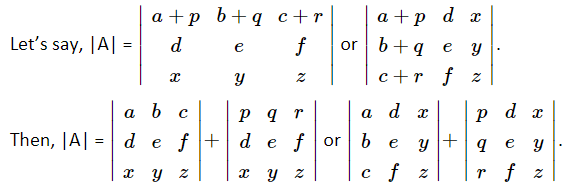## Invariance Property of Determinants

The invariance properties of determinants determines that if each element of a row (or column) of a determinant is multiplied by the same constant and then added to the corresponding elements of some other row (or column), then the value of the determinant remains the same.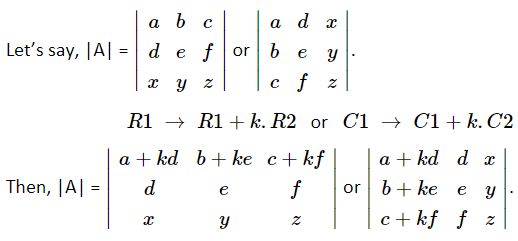This is also known as Elementary Transformation.

## Triangle Property of Determinants

The triangle property of determinants utters that if each element of a determinant above or below the main diagonal comprises of zeros, then the determinant is equal to the product of all the diagonal elements.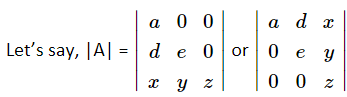. Then, |A| = aez.

## Diagonal Property of Determinants

The diagonal property of determinants is somehow a bit similar to the triangle property of determinants. It indicates that if all the other element of a determinant are 0 except the diagonal elements only, then the determinant is equal to the product of all the diagonal elements.

Let’s say,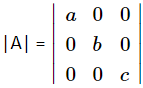. Then, |A| = abc.

Simply, it is said that if A = [aij] is a diagonal matrix of order n (or A = diag (a, b, c,…)), then |A| is equal to the product of the diagonal elements,

## Co-Factor Properties of Determinants

The co-factor properties of determinants can be described as follows:

• The determinant of the matrix A is the same as the determinant of the co-factors of all the elements of matrix A, i.e.  Let’s say,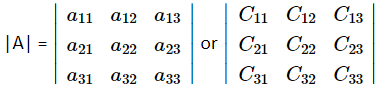; where, Cij = The Co-Factors of the elements aij in the determinant.
• Let A be a square matrix of order n, then the sum of the product of elements of any row (or column) with their co-factors is always equal to the |A|.
• a11C11 + a12C12 + a13C13 = |A|.
• a11C11 + a21C21 + a31C31 = |A|.

## Singular Property of Determinants

A square matrix A is said to singular, if and only if (iff) the value of determinant is 0, i.e. |A| = 0.

## Non-Singular Property of Determinants

A square matrix A is said to non-singular, if and only if (iff) the value of determinant is not equal to 0, i.e. |A| ≠ 0.

## Picked For You

Furthermore, these are some topics which might interest you: